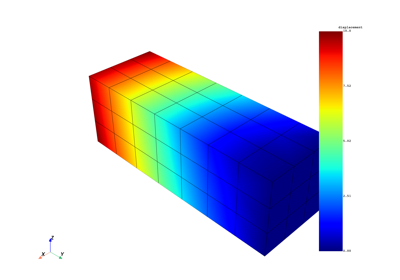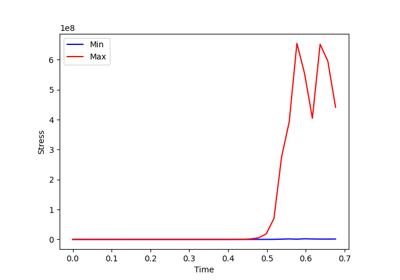These examples show advanced use cases to demonstrate the high level of workflow customization.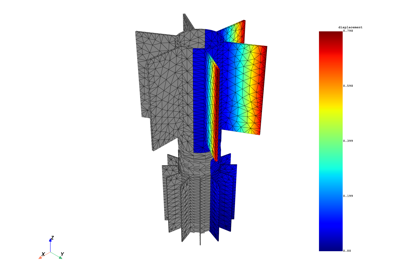Multi-stage cyclic symmetry using advanced customization

Multi-stage cyclic symmetry using advanced customization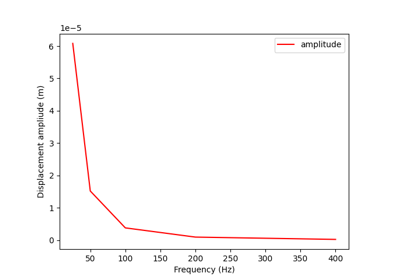Solve harmonic problem (with damping) using matrix inverse

Solve harmonic problem (with damping) using matrix inverse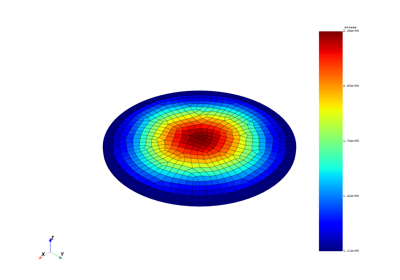Average elemental stress on a given volume

Average elemental stress on a given volume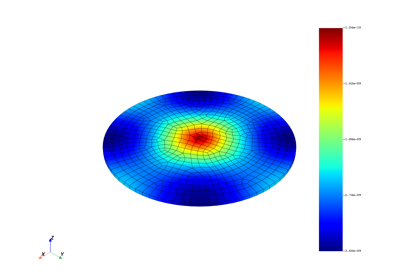Exchange data between servers

Exchange data between servers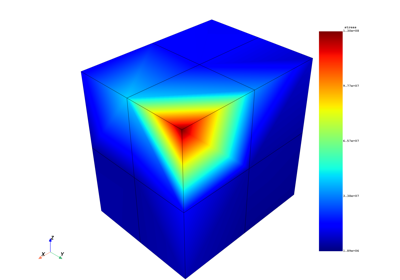Extrapolation method for stress result of a 3D element

Extrapolation method for stress result of a 3D element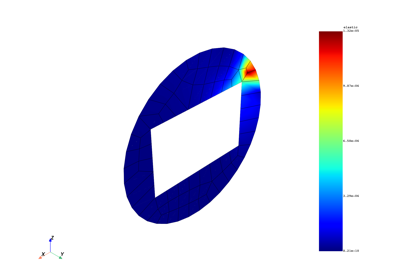Extrapolation method for strain result of a 2D element

Extrapolation method for strain result of a 2D element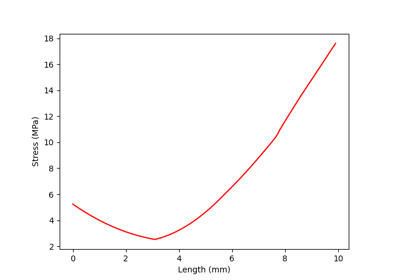Stress gradient normal to a defined node

Stress gradient normal to a defined node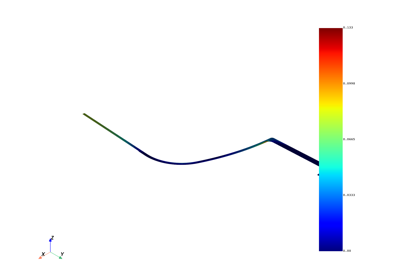Pressure vessel analysis according to an ASME standard

Pressure vessel analysis according to an ASME standard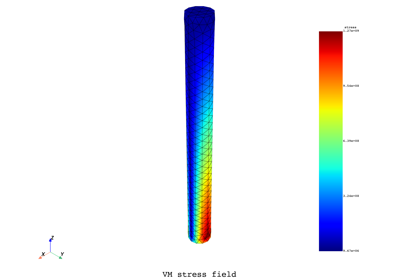Calculate the number of cycles to fatigue failure

Calculate the number of cycles to fatigue failure